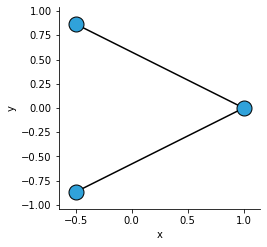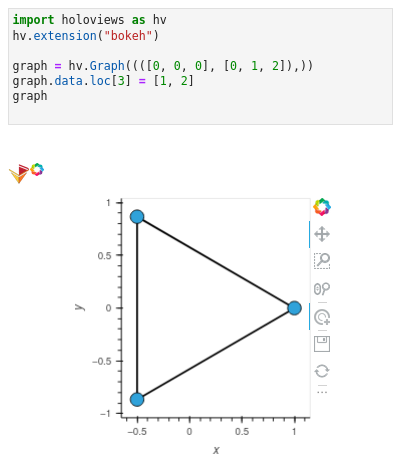# Add an edge to a graph after it has been created

Hello everyone,

how do I add edges to an holoviews graph after it has been constructed? Is there something like `graph.add_edge()`?

I have prepared a minimal working example below, where I want to connect the remaining two nodes with an edge (so that all nodes are connected) after the graph has already been created.

``````import holoviews as hv

graph = hv.Graph((([0, 0, 0], [0, 1, 2]),)) # produces a small graph
# The edge connecting the last two nodes should be added after this line
#
hv.save(graph, "graph_with_missing_edge.png")
``````I ask because I‘m working on a program where the base graph has already been created and depending on the interactive parameters in a dynamic map, edges have to be added later on.

As a followup question:
How would this work if the added edge should be a parallel edge (where one connecting edge already exists, but a second should be added)? If it looks more natural, the original edge can be edited too when the parallel edge is inserted. (I would like that process to work multiple times on the same pair of nodes/ the same edge).

If you have any ideas on how to do this, please let me know, I would really appreciate it. Thanks in advance!

You can do something like this:1 Like

Thank you, that worksDo you have any idea how I can add parallel edges that way (or any other way)? For example, something like `graph.data.loc = [1, 2]` will just draw over the already existing edge.

Hi @optmat

Could you please open a new post with the new question including a minimum, reproducible example.

This makes it much easier for the community to search, follow and interact with as the discussion grows. Thanks.

1 Like

Thanks for the advice. I’ve done so in https://discourse.holoviz.org/t/create-parallel-edges/4107.